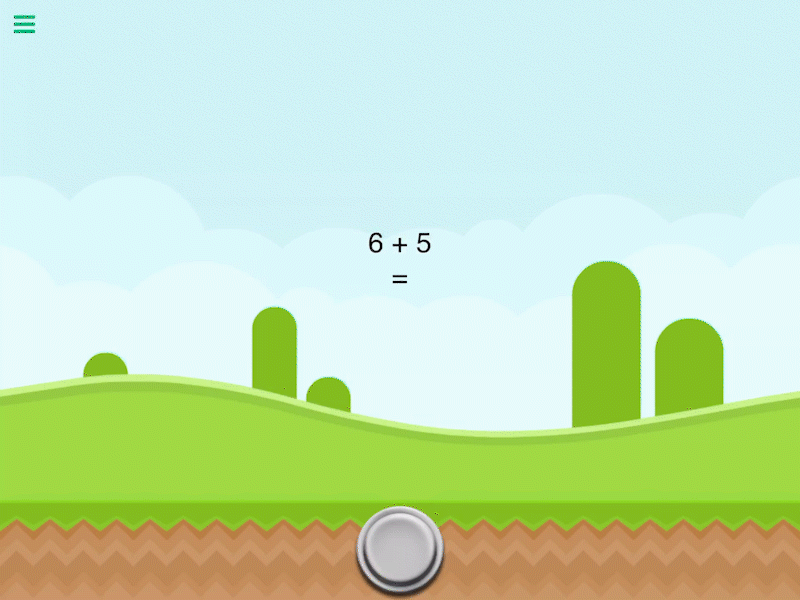Description

Add 2 values together and output their sum.

Outputs

 Add Values Outputs the sum of two inputs.

Examples

2 added to 2 outputs 4.

Related

Divide Values

Modulus

Multiply Values

Square Root